Fault Location in Underground Cables using ANFIS Nets and Discrete Wavelet Transform | OMICS International
Journal of Electrical & Electronic Systems

# Fault Location in Underground Cables using ANFIS Nets and Discrete Wavelet Transform

Barakat S1*, Eteiba MB2 and Wahba WI2

1Electrical Engineering Department, Beni Suief University, Beni Suief, Egypt

2Electrical Engineering Department, Fayoum University, Fayoum, Egypt

*Corresponding Author:
Barakat S
Electrical Engineering Department
Beni Suief University, Beni Suief, Egypt
Tel: +20 01115153369
E-mail: [email protected]

Received Date: May 12, 2014; Accepted Date: July 16, 2014; Published Date: July 29, 2014

Citation: Barakat S, Eteiba MB, Wahba WI (2014) Fault Location in Underground Cables using ANFIS Nets and Discrete Wavelet Transform. J Electr Electron Syst 3:129. doi:10.4172/2332-0796.1000129

Copyright: © 2014 Barakat S, et al. This is an open-access article distributed under the terms of the Creative Commons Attribution License, which permits unrestricted use, distribution, and reproduction in any medium, provided the original author and source are credited.

Visit for more related articles at Journal of Electrical & Electronic Systems

#### Abstract

This paper presents an accurate algorithm for locating faults in a medium voltage underground power cable using Adaptive Network-Based Fuzzy Inference System (ANFIS). The proposed method uses five ANFIS networks and consists of 2 stages, including fault type classification and exact fault location. In the first part, an ANFIS is used to determine the fault type, applying four inputs, i.e., the maximum detailed energy of three phase and zero sequence currents. Other four ANFIS networks are utilized to pinpoint the faults (one for each fault type). Four inputs, i.e., the maximum detailed energy of three phase and zero sequence currents, are used to train the Neuro-fuzzy inference systems in order to accurately locate the faults on the cable. The proposed method is evaluated under different fault conditions such as different fault locations, different fault inception angles and different fault resistances.

#### Keywords

ANFIS; Fault location; Underground cable; Wavelet Transform

#### Introduction

Underground cables have been widely implemented due to their reliability and limited environmental concerns. To improve the reliability of a distribution system, accurate identification of a faulted segment is required in order to reduce the interruption time during fault. Therefore, a rapid and accurate fault detection method is required to accelerate system restoration, reduce outage time, minimize financial losses and significantly improve the system reliability.

Various fault location algorithms for underground cables have been developed so far. For example, Ningkang and Yuan introduced a mathematical model that is based on calculating the impedance across a tested transmission line to localize all fault locations .

Although their model was satisfactory, they only used the postfault phase magnitude current to identify fault location; however, their method is not applicable to the distribution system due to asymmetrical network. An alternative approach to identify fault location for a radial cable employed wavelet transform to extract valuable information from transient signals and eventually localize faults through a fuzzy logic system is presented in . Javad implemented another approach locate faults in a combined overhead transmission line with underground power cable using ANFIS . Wavelet transform is used to obtain the current patterns in ; the proposed methodology consists on training the ANFIS system with a fault database registers obtained from the power distribution system. The performance of the ANFIS nets was good and the 99.14% of the current patterns were correctly classified.

Here, we build upon previously presented methods and describe a fast and accurate method that to detect fault location in underground cables. The proposed method uses a novel wavelet-ANFIS combined approach. The ANFIS is used to extract information from the available Discrete Wavelet Transform coefficients to obtain coherent conclusions regarding fault location. Similar to any rule-based system, the rules are gathered through a Fuzzy Inference System (FIS) . The efficacy of the proposed model was validated under different fault conditions.

This paper is organized as follows. Section 2, provides a brief introduction to wavelet transform. The theoretical background on ANFIS is presented in section 3, while the methodology that was used to locate faults is presented in section 4. The training and testing results are presented in section 5 followed by conclusions in section 6.

#### Wavelet Transform Analysis

Recently, Wavelet Transform (WT) techniques have been effectively used for multi-scale representation and analysis of many signals . A wavelet transform decomposes transients into a series of wavelet components, each of which corresponds to a time-domain signal that covers a specific frequency band that contains more detailed information. Wavelets localize the information in the time–frequency plane; in particular, by exchanging one type of resolution by another. This makes wavelet transform techniques more suited for analyzing non-stationary signals. Wavelet analysis is also appropriate for rapid changes in transient signal analysis. Its major strength is its ability to demonstrate the local features of a particular area of a large signal. WT divides up data, operates for different frequency components, and then studies each component with a resolution that is matched to its scale .

Theory of discrete wavelet transform

In a Discrete Wavelet Transform (DWT), a time-scale representation of a digital signal is obtained using digital filtering techniques. In this case, filters of different cut-off frequencies are used to analyze the signal at different scales . The signal then passes through a series of high pass filters to analyze the high frequencies, and through a series of low pass filters to analyze its low frequency components. Hence the signal (S) will be decomposed into two types of components, namely an approximation (termed A) and a detail (D). The approximation is the high scale, low-frequency component of the signal while the detail is the low-scale, high-frequency components. This decomposition process can be iterated, using successive approximations which can be further decomposed such that any given signal is broken down into many lower resolution components. This approach is called the wavelet decomposition tree which is shown in Figure 1.

#### The ANFIS Concept

ANFIS is a simple data learning technique that adopts a fuzzy inference system model to transform a given input into a target output. This transformation involves membership functions, fuzzy logic operators and conditional rules. ANFIS is a Sugeno model from a development of Fuzzy Inference System (FIS) [9,10]. Sugeno-type ANFIS implements a first-order polynomial to the output system in order to replace zero-order in Sugeno FIS model. There are five main processing stages in a typical ANFIS operation, which include input fuzzification, application of fuzzy operators, application method, and output aggregation and defuzzification . These stages are demonstrated in the ANFIS structure as shown in Figure 2. Each ANFIS layer has specific functions for calculating input and output parameter sets as described below .

Layer 1: Fuzzification of input data is performed in this layer. The following equations are used for this process: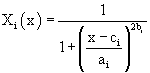(1)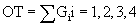(2)

Where, Xi(x) and Yi(y) are fuzzied values, and ai, bi and ci are the parameters sets that are calculated by Gaussian input membership function.

Layer 2: This layer involves fuzzy operators and it uses the product (AND) operator to fuzzify the inputs. The following fuzzy relationship represents the products of fuzzy operators: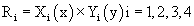(3)

Layer 3 (Normalization): In this stage, every gained signal is divided to the total of gained signal by the following equation,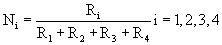(4)

Layer 4 (Defuzzification): In this stage, a normalized signal is gained again through a linear equation that is formed from the membership function of the output signal as shown in the following equation,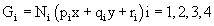(5)

Where, pi, qi and ri being the membership function parameters for the linear signal.

Layer 5: The last process in the ANFIS operation is called neuron addition in which all defuzzification signals, Gi are added together as shown below:(6)

OT is a predicted value.

#### Proposed Fault Location Method

The proposed method consists of two main stages namely, fault type classification and exact fault location. The presented algorithm contains five ANFISs. The first network is for fault type classification, while the remaining four networks are for accurate fault location (one for each fault type).

Features extraction using DWT

Here, we used the line current signals as the input to the DWT. Daubechies DB4 wavelet, is employed since it has been demonstrated to high performance. The fault transients of the study cases are analyzed through discrete wavelet transform at the level five. Both approximation and details information related fault current are extracted from the original signal with the multi-resolution analysis.

When a fault occurs in the cable, its effect can be observed as variations within the decomposition coefficient of the current signals that contain useful fault signatures. Figure 3 shows the DWT detailed coefficients at level 1 to level 5 for a particular type of fault studied in the work. The nature of the plot of detailed coefficients at level 1 reveals a sharp spike which corresponds to the fault initiation process. According to DWT theory, this spike represents the highest frequency within the fault signal. It is however, not practical to identify a fault based on this spike only since such spikes will occur every time there is a sudden change in the cable current signal. This will thus not be able to clearly differentiate between faults of different types and at different locations.

Figure 3: The DWT detailed coefficients at level 1 to level 5 for a three phase to ground fault (phase A).

The nature of level 5 detailed coefficients (Figures 4 and 5) shows that along with the high spike, there is a certain side band containing some smaller spikes. The nature of this side band along with the dominant spike has been observed to change appreciably with variations in fault types and locations. Detailed coefficients at still higher levels, however, have been found to contain much wider side bands that complicated correlation with possible fault types and locations.

Therefore, we decided to start with, extracting some meaningful features from the level 5 detailed coefficients that can be correlated to possible fault types and locations. With this in mind, the maximum detailed energy of three phase and zero sequence currents have been used as features of the fault classification and location scheme. The proposed methodology consists of training the ANFIS system with a fault database obtained from the simulation of the cable.

Fault classification scheme

In order to design an ANFIS, it is crucial to train it efficiently and correctly. The training set must be carefully chosen such that it can include a diversity of fault conditions such as different fault inception angles, different fault resistances and different fault locations are considered. The performance of the ANFIS is then tested using both patterns within and outside of the training set.

An acceptable and simple criterion that we used here is that the ANFIS input should provide more information for fault location than those not selected. Therefore, for fault type classification (ANFIS1), the maximum detailed energy of three phases and zero sequence currents are selected as inputs and the desired output is the fault type as set in Table 1.

Fault Type ANFIS Target
Three Phase to ground (ABCG) 1
Double line to ground (ABG) 2
line to line (AB) 3
Single line to ground (AG) 4

Table 1: Training target for ANFIS1 fault type classification.

Fault location scheme

In this stage, four different ANFISs are trained for fault location based on the knowledge of the fault type. Once the fault is classified, the relevant ANFIS for fault location is activated. The inputs for these networks are the same as those for the inputs of ANFIS1. The output, however, is the distance of the fault point from the sending end of the cable in Km.

#### Tests and Results

Test system

The Alternative Transient Program (ATP) is used to simulate medium voltage underground cable model  with a sampling frequency of 200 KHz. The single line diagram is shown in Figure 6. The components that we used are the three phase voltage source, a tested cable and a fixed load. The specifications of cable material for 11 Kv are presented in Table 2.

Specification of MV underground cable material ( XLPE Stranded Copper Conductor - 6 Km - Bergeron model )
r3=12.05
r4=12.2, r5=13.8
Core conductor r=1.7 E-8 Ω.m ,µ=1.0
Insulation µ=1.0,e=2.7
Sheath r=2.5 E-8 Ω.m, µ=1.0

Table 2: Specification of Cable material for 11 Kv

Three phase voltage source: V=11KV with f=50 Hz

Load: Three-Phase 2 MVA Grounded-Wye load with parallel R, L elements (power factor=0.85, R=71.157 Ω, L=365.475mH).

Simulation of MV underground cable faults depends on four main fault parameters (fault type, fault distance, fault resistance, inception angle).

Training scenarios for the simulation

Fault type

• Single line to ground (AG)

• Double line to ground (ABG)

• Line to line (AB)

• Three phase to ground

Fault resistance: {0, 10, 30, 50, 100, 200 Ω}

Inception angle: {0°, 45°, 90°, 135°, 180°}

Fault distance:

• Training: [0.5, 0.75, 1, 1.25, 1.5, 1.75, 2, 2.25, 2.5, 2.75, 3, 3.25, 3.5, 3.75, 4, 4.25, 4.5, 4.75, 5, 5.25, 5.5] Km from the sending end.

Testing: [0.625, 0.875, 1.125, 1.375, 1.625, 1.875, 2.125, 2.375, 2.625, 2.875, 3.125, 3.375, 3.625, 3.875, 4.125, 4.375, 4.625, 4.875, 5.125, 5.375] Km from the sending end.

Table 3 shows the number of simulations used in this work.

Training Testing
Three phase to ground 630 120
Single line to ground (AG) 630 180
Line to line (AB) 630 120
Double line to ground (ABG) 630 120
Total 2520 540

Table 3: Number of simulations.

Fault data base

To obtain a fault data-base, the four fault types were simulated in the MV underground cable shown in Figure 5: Single phase-fault to ground (Fault phase A to ground, Line to line fault (Fault phase A and B), Double line to ground fault (Fault phase A and B to ground) and three-phase to ground fault (fault phases A, B, C to ground). The simulated ATP files are then converted to a Microsoft Office Excel Comma Separated Values File (*.csv file) and imported to Matlab to perform wavelet analysis and the extracted features are stored in a database file. As a result 3060 simulations have been obtained. These were used to train and validate the ANFIS system.

ANFIS fault type classification results

Table 4 lists part of the fault classification training output results for all types of fault. As shown, the obtained predicted values are quite similar to that of the training target values. This demonstrates that ANFIS is able to recognize and classify fault correctly.

Fault Type ANFIS Output Fault Type ANFIS Output Fault Type ANFIS Output Fault Type ANFIS Output
ABCG 1.00 ABG 1.88 AB 3.01 AG 4.01
ABCG 1.00 ABG 1.88 AB 3.01 AG 4.01
ABCG 1.00 ABG 1.98 AB 3.01 AG 4.01
ABCG 0.94 ABG 2.00 AB 3.00 AG 4.00
ABCG 0.99 ABG 2.00 AB 3.00 AG 4.00
ABCG 1.00 ABG 2.00 AB 3.00 AG 4.01
ABCG 0.99 ABG 1.99 AB 3.00 AG 4.01
ABCG 1.00 ABG 2.01 AB 3.00 AG 4.03
ABCG 0.99 ABG 1.99 AB 3.00 AG 4.01
ABCG 1.00 ABG 2.01 AB 3.00 AG 4.03
ABCG 1.01 ABG 2.01 AB 3.00 AG 4.00
ABCG 1.02 ABG 2.00 AB 2.99 AG 4.00
ABCG 1.02 ABG 2.00 AB 3.01 AG 4.01
ABCG 1.02 ABG 2.00 AB 3.00 AG 4.01
ABCG 1.02 ABG 2.01 AB 2.99 AG 4.00
ABCG 1.16 ABG 2.03 AB 2.99 AG 4.01
ABCG 1.01 ABG 2.00 AB 3.02 AG 4.01
ABCG 1.01 ABG 2.03 AB 3.00 AG 4.00
ABCG 1.00 ABG 1.99 AB 3.00 AG 4.03
ABCG 1.00 ABG 1.97 AB 3.01 AG 4.00

Table 4: The fault classification training output results for all types of fault.

Fault location system tool

Development of the fault location system tool (FL) is based on Matlab GUI as shown in Figure 7. The purposes of building the GUI tool are to:

• Test the data using ANFIS models,

• Display the original signal, the wavelet approximation and detail coefficients at level 5,

• Produces fault types,

• Calculate fault location and

• Calculate the percentage error.

The FL needs only a standard data format of three-phase current as an input to pin point the fault.

ANFIS fault location estimation training results

In ANFIS Fault Location Estimation, all the four types of fault (ABCG, ABG, AB and AG) were trained separately. As a result, a total of four networks were used to estimate the fault distance. The results for each network are presented below.

The location error is defined as :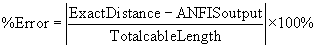(6)

where ,Total cable Length=6 Km

Three phase to ground ANFIS fault location model: It can be noticed from Figure 8 that the Percentage error of three phases to ground fault is less than 1.65%.

Double line to ground ANFIS fault location model: It can be noticed from Figure 9 that the Percentage error of double Line to ground fault is less than 1%.

Line to line ANFIS fault location model: It can be noticed from Figure 10 that the Percentage error of Line to Line fault is less than 2%.

Single line to ground ANFIS fault location model: It can be noticed from Figure 11 that the Percentage error of Single Line to ground fault is less than 3%.

ANFIS fault location estimation testing results

Three phase to ground fault: It can be noticed from Figure 12 that the Percentage error of three phases to ground fault is less than 0.7%.

Double line to ground fault: It can be noticed from Figure 13 that the Percentage error of double Line to ground fault is less than 1.7%.

Line to line fault: It can be noticed from Figure 14 that the Percentage error of Line to Line fault is less than 1.8%.

Single Line to ground fault: It can be noticed from Figure 15 that the Percentage error of Single Line to ground fault is less than 3%.

#### Conclusion

This paper presented an application of fault location method to localize faults in a medium voltage underground cable based on the theory of Wavelet and Adaptive Network Fuzzy Inference System (ANFIS). The Proposed ANFIS uses only post-fault three-phase currents as inputs. It predicts the distance of the fault from the sending end point. The results show that the approach can accurately identify the faults types and locate the faults.

#### References

Select your language of interest to view the total content in your interested language

### Article Usage

• Total views: 12488
• [From(publication date):
December-2014 - Dec 08, 2019]
• Breakdown by view type
• HTML page views : 8672Can't read the image? click here to refresh